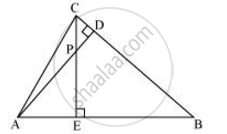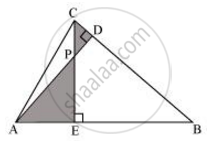Share

# In the following figure, altitudes AD and CE of ΔABC intersect each other at the point P. Show that - CBSE Class 10 - Mathematics

ConceptCriteria for Similarity of Triangles

#### Question

In the following figure, altitudes AD and CE of ΔABC intersect each other at the point P. Show that:ΔAEP ∼ ΔCDP

#### SolutionIn ΔAEP and ΔCDP,

∠AEP = ∠CDP (Each 90°)

∠APE = ∠CPD (Vertically opposite angles)

Hence, by using AA similarity criterion,

ΔAEP ∼ ΔCDP

Is there an error in this question or solution?

#### APPEARS IN

NCERT Solution for Mathematics Textbook for Class 10 (2019 to Current)
Chapter 6: Triangles
Ex. 6.30 | Q: 7.1 | Page no. 140

#### Video TutorialsVIEW ALL 

Solution In the following figure, altitudes AD and CE of ΔABC intersect each other at the point P. Show that Concept: Criteria for Similarity of Triangles.
S